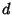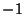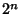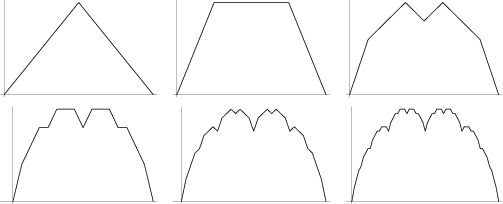## Blancmange FunctionA Continuous Function which is nowhere Differentiable. The iterations towards the continuous function are Batrachions resembling the Hofstadter-Conway $10,000 Sequence. The first six iterations are illustrated below. Theth iteration containspoints, where, and can be obtained by setting, lettingand looping overto 1 by steps ofandtoby steps of.Peitgen and Saupe (1988) refer to this curve as the Takagi Fractal Curve. See also Hofstadter-Conway$10,000 Sequence, Weierstraß Function

References

Dixon, R. Mathographics. New York: Dover, pp. 175-176 and 210, 1991.

Peitgen, H.-O. and Saupe, D. (Eds.). Midpoint Displacement and Systematic Fractals: The Takagi Fractal Curve, Its Kin, and the Related Systems.'' §A.1.2 in The Science of Fractal Images. New York: Springer-Verlag, pp. 246-248, 1988.

Takagi, T. A Simple Example of the Continuous Function without Derivative.'' Proc. Phys. Math. Japan 1, 176-177, 1903.

Tall, D. O. The Blancmange Function, Continuous Everywhere but Differentiable Nowhere.'' Math. Gaz. 66, 11-22, 1982.

Tall, D. The Gradient of a Graph.'' Math. Teaching 111, 48-52, 1985.